/Matplotlib 3.1

# matplotlib.pyplot.hist

`matplotlib.pyplot.hist(x, bins=None, range=None, density=None, weights=None, cumulative=False, bottom=None, histtype='bar', align='mid', orientation='vertical', rwidth=None, log=False, color=None, label=None, stacked=False, normed=None, *, data=None, **kwargs)` [source]

Plot a histogram.

Compute and draw the histogram of x. The return value is a tuple (n, bins, patches) or ([n0, n1, ...], bins, [patches0, patches1,...]) if the input contains multiple data. See the documentation of the weights parameter to draw a histogram of already-binned data.

Multiple data can be provided via x as a list of datasets of potentially different length ([x0, x1, ...]), or as a 2-D ndarray in which each column is a dataset. Note that the ndarray form is transposed relative to the list form.

Masked arrays are not supported at present.

Parameters: `x : (n,) array or sequence of (n,) arrays` Input values, this takes either a single array or a sequence of arrays which are not required to be of the same length. `bins : int or sequence or str, optional` If an integer is given, `bins + 1` bin edges are calculated and returned, consistent with `numpy.histogram`. If `bins` is a sequence, gives bin edges, including left edge of first bin and right edge of last bin. In this case, `bins` is returned unmodified. All but the last (righthand-most) bin is half-open. In other words, if `bins` is: ```[1, 2, 3, 4] ``` then the first bin is `[1, 2)` (including 1, but excluding 2) and the second `[2, 3)`. The last bin, however, is `[3, 4]`, which includes 4. Unequally spaced bins are supported if bins is a sequence. With Numpy 1.11 or newer, you can alternatively provide a string describing a binning strategy, such as 'auto', 'sturges', 'fd', 'doane', 'scott', 'rice' or 'sqrt', see `numpy.histogram`. The default is taken from `rcParams["hist.bins"]`. `range : tuple or None, optional` The lower and upper range of the bins. Lower and upper outliers are ignored. If not provided, range is `(x.min(), x.max())`. Range has no effect if bins is a sequence. If bins is a sequence or range is specified, autoscaling is based on the specified bin range instead of the range of x. Default is `None` `density : bool, optional` If `True`, the first element of the return tuple will be the counts normalized to form a probability density, i.e., the area (or integral) under the histogram will sum to 1. This is achieved by dividing the count by the number of observations times the bin width and not dividing by the total number of observations. If stacked is also `True`, the sum of the histograms is normalized to 1. Default is `None` for both normed and density. If either is set, then that value will be used. If neither are set, then the args will be treated as `False`. If both density and normed are set an error is raised. `weights : (n, ) array_like or None, optional` An array of weights, of the same shape as x. Each value in x only contributes its associated weight towards the bin count (instead of 1). If normed or density is `True`, the weights are normalized, so that the integral of the density over the range remains 1. Default is `None`. This parameter can be used to draw a histogram of data that has already been binned, e.g. using `np.histogram` (by treating each bin as a single point with a weight equal to its count) ```counts, bins = np.histogram(data) plt.hist(bins[:-1], bins, weights=counts) ``` (or you may alternatively use `bar()`). `cumulative : bool, optional` If `True`, then a histogram is computed where each bin gives the counts in that bin plus all bins for smaller values. The last bin gives the total number of datapoints. If normed or density is also `True` then the histogram is normalized such that the last bin equals 1. If cumulative evaluates to less than 0 (e.g., -1), the direction of accumulation is reversed. In this case, if normed and/or density is also `True`, then the histogram is normalized such that the first bin equals 1. Default is `False` `bottom : array_like, scalar, or None` Location of the bottom baseline of each bin. If a scalar, the base line for each bin is shifted by the same amount. If an array, each bin is shifted independently and the length of bottom must match the number of bins. If None, defaults to 0. Default is `None` `histtype : {'bar', 'barstacked', 'step', 'stepfilled'}, optional` The type of histogram to draw. 'bar' is a traditional bar-type histogram. If multiple data are given the bars are arranged side by side. 'barstacked' is a bar-type histogram where multiple data are stacked on top of each other. 'step' generates a lineplot that is by default unfilled. 'stepfilled' generates a lineplot that is by default filled. Default is 'bar' `align : {'left', 'mid', 'right'}, optional` Controls how the histogram is plotted. 'left': bars are centered on the left bin edges. 'mid': bars are centered between the bin edges. 'right': bars are centered on the right bin edges. Default is 'mid' `orientation : {'horizontal', 'vertical'}, optional` If 'horizontal', `barh` will be used for bar-type histograms and the bottom kwarg will be the left edges. `rwidth : scalar or None, optional` The relative width of the bars as a fraction of the bin width. If `None`, automatically compute the width. Ignored if histtype is 'step' or 'stepfilled'. Default is `None` `log : bool, optional` If `True`, the histogram axis will be set to a log scale. If log is `True` and x is a 1D array, empty bins will be filtered out and only the non-empty `(n, bins, patches)` will be returned. Default is `False` `color : color or array_like of colors or None, optional` Color spec or sequence of color specs, one per dataset. Default (`None`) uses the standard line color sequence. Default is `None` `label : str or None, optional` String, or sequence of strings to match multiple datasets. Bar charts yield multiple patches per dataset, but only the first gets the label, so that the legend command will work as expected. default is `None` `stacked : bool, optional` If `True`, multiple data are stacked on top of each other If `False` multiple data are arranged side by side if histtype is 'bar' or on top of each other if histtype is 'step' Default is `False` `normed : bool, optional` Deprecated; use the density keyword argument instead. `n : array or list of arrays` The values of the histogram bins. See density and weights for a description of the possible semantics. If input x is an array, then this is an array of length nbins. If input is a sequence of arrays `[data1, data2,..]`, then this is a list of arrays with the values of the histograms for each of the arrays in the same order. The dtype of the array n (or of its element arrays) will always be float even if no weighting or normalization is used. `bins : array` The edges of the bins. Length nbins + 1 (nbins left edges and right edge of last bin). Always a single array even when multiple data sets are passed in. `patches : list or list of lists` Silent list of individual patches used to create the histogram or list of such list if multiple input datasets. `**kwargs : Patch properties`

`hist2d`
2D histograms

#### Notes

Note

In addition to the above described arguments, this function can take a data keyword argument. If such a data argument is given, the following arguments are replaced by data[<arg>]:

• All arguments with the following names: 'weights', 'x'.

Objects passed as data must support item access (`data[<arg>]`) and membership test (`<arg> in data`).

## Examples using `matplotlib.pyplot.hist`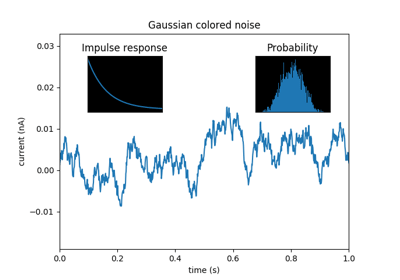Axes Demo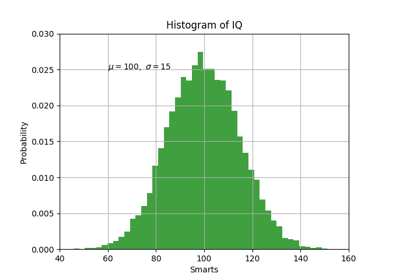Pyplot Text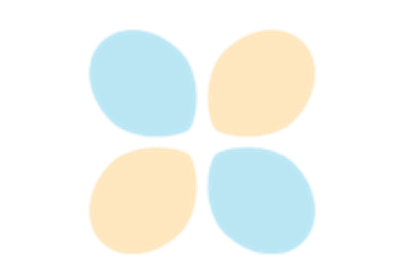SVG Histogram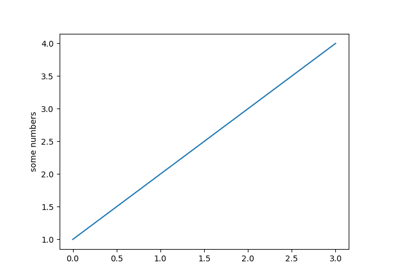Pyplot tutorial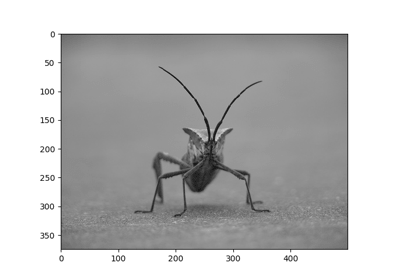Image tutorial